Author: Oscar Cronquist Article last updated on February 13, 2023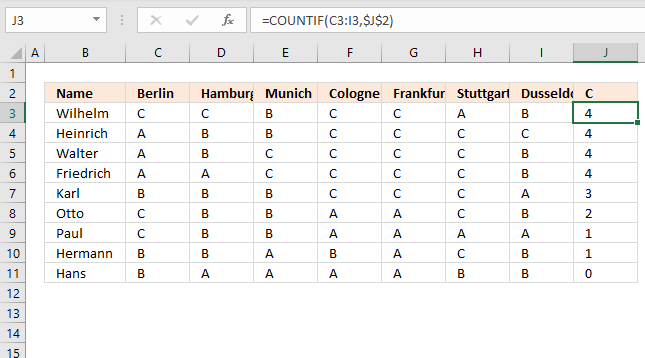In this article, I will demonstrate two techniques for counting per row. The first example is simple and straightforward.

The second example is a bit more complicated but dynamic and automatic, you only need to provide a search string, everything else is calculated by array formulas.

## 1. Count value per row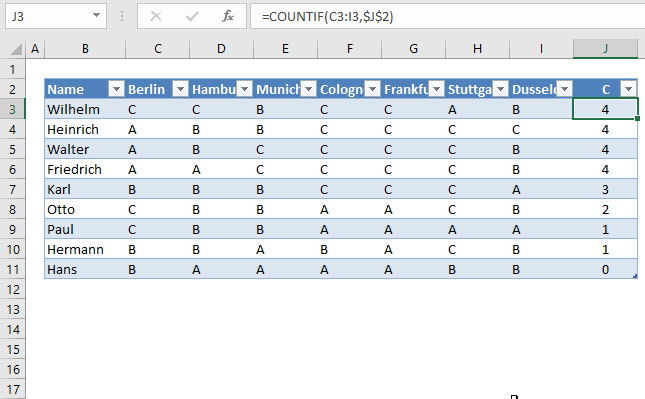The first example has this data table, see image above. It is an Excel Table, select any cell in the data set and press CTRL + T to convert the data to an Excel Table.

I want to count the value "C" per row.
In cell J3, type:

=COUNTIF(C3:I3, \$J\$2)

Copy cell I2 and paste to I3:I10. You don't need to do this if you use an Excel Table as I did, see the image above. The Excel Table copies the formulas to cells below automatically.

The COUNTIF function counts the number of cells that meet a given condition.

=COUNTIF(range, criteria)

There are 4 C's in row 2 so the formula returns 4 in cell J3. If you change the value in cell J2 to "B", the formulas in J3:J11 recalculates and return new values.

Let us sort the Excel table.Drop-down list arrows appear next to each header, see picture above. Press with left mouse button on the arrow next to "C".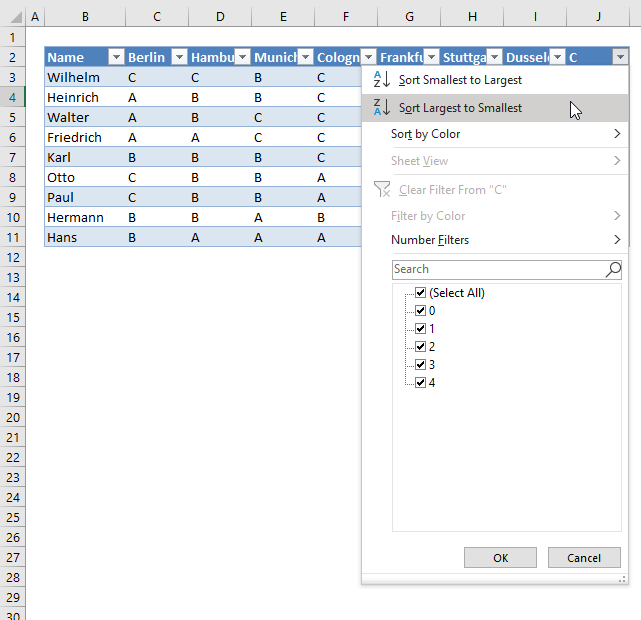Press with left mouse button on "Largest to smallest". The data table shows the count of "C" per row, sorted largest to smallest.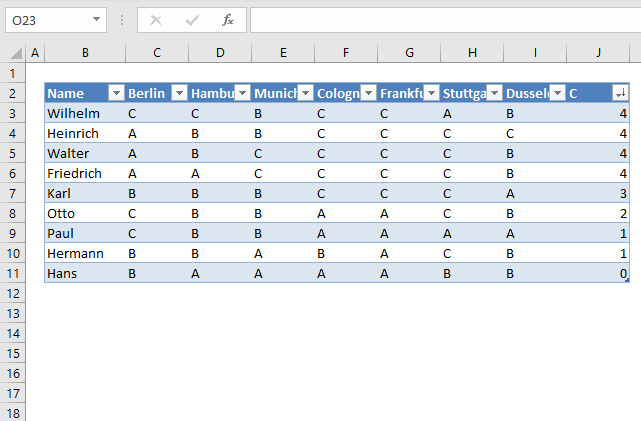Do you know why \$I\$1 in COUNTIF(B2:H2,\$I\$1) has dollar signs?
Read this post: Absolute and relative cell references

## 2. Dynamic counting per row sorted from large to small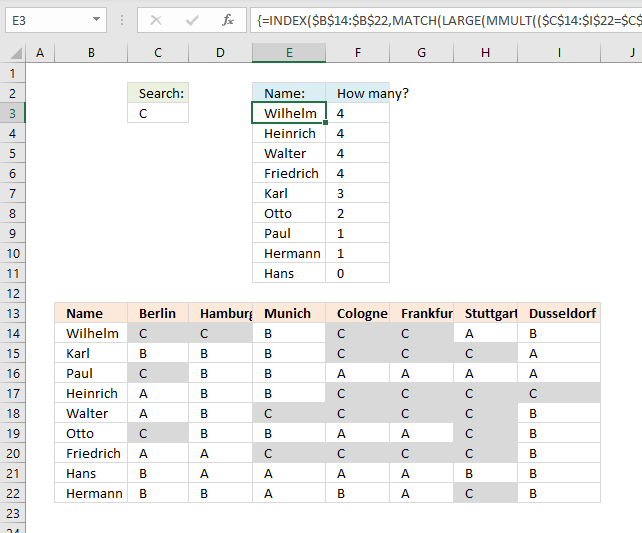Now on to a more interesting and complicated example. The formula here returns names sorted in column E based on the number of "C"s per row.

How is this possible? The MMULT function is able to count cells per row based on a condition, it returns an array that corresponds to the number of rows. Change the value in cell C3 and the list in cell range E3:F11 is instantly changed.

I have applied conditional formatting to cell range B14:I22 so you can easily verify the calculated numbers in E3:E11.

Array formula in cell E3:

=INDEX(\$B\$14:\$B\$22, MATCH(LARGE(MMULT((\$C\$14:\$I\$22=\$C\$3)*1, TRANSPOSE(COLUMN(\$C\$14:\$I\$22)^0)), ROWS(\$B\$1:B1)), IF(COUNTIF(\$E\$2:\$E2, \$B\$14:\$B\$22)=0, MMULT((\$C\$14:\$I\$22=\$C\$3)*1, TRANSPOSE(COLUMN(\$C\$14:\$I\$22)^0)), ""), 0))

Excel 365 dynamic array formula in cell E3:

=SORTBY(B14:B22, MMULT((\$C\$14:\$I\$22=\$C\$3)*1, TRANSPOSE(COLUMN(\$C\$14:\$I\$22)^0)), -1)

The formula above works only in Excel 365, it contains a new function: SORTBY.

Array formula in cell E3:

=LARGE(MMULT((\$B\$14:\$H\$22=\$B\$3)*1, TRANSPOSE(COLUMN(\$B\$14:\$H\$22)^0)), ROWS(\$A\$1:A1))

### Explaining array formula in cell E3

#### Step 1 - Find search value in cell range

The equal sign compares the value in cell C3 to values in cell range c14:I22.

\$C\$14:\$I\$22=\$C\$3

becomes

{"C", "C", "B", "C", "C", "A", "B";"B", "B", "B", "C", "C", "C", "A";"C", "B", "B", "A", "A", "A", "A";"A", "B", "B", "C", "C", "C", "C";"A", "B", "C", "C", "C", "C", "B";"C", "B", "B", "A", "A", "C", "B";"A", "A", "C", "C", "C", "C", "B";"B", "A", "A", "A", "A", "B", "B";"B", "B", "A", "B", "A", "C", "B"}="C"

and returns

{TRUE, TRUE, FALSE, TRUE, TRUE, FALSE, FALSE;FALSE, FALSE, FALSE, TRUE, TRUE, TRUE, FALSE;TRUE, FALSE, FALSE, FALSE, FALSE, FALSE, FALSE;FALSE, FALSE, FALSE, TRUE, TRUE, TRUE, TRUE;FALSE, FALSE, TRUE, TRUE, TRUE, TRUE, FALSE;TRUE, FALSE, FALSE, FALSE, FALSE, TRUE, FALSE;FALSE, FALSE, TRUE, TRUE, TRUE, TRUE, FALSE;FALSE, FALSE, FALSE, FALSE, FALSE, FALSE, FALSE;FALSE, FALSE, FALSE, FALSE, FALSE, TRUE, FALSE}

#### Step 2 - Convert boolean values to numerical values

The parentheses lets you control the order of calculation, we want to compare \$B\$14:\$H\$22 with \$B\$3 before we multiply with 1.

(\$B\$14:\$H\$22=\$B\$3)*1

becomes

{TRUE, TRUE, FALSE, TRUE, TRUE, FALSE, FALSE;FALSE, FALSE, FALSE, TRUE, TRUE, TRUE, FALSE;TRUE, FALSE, FALSE, FALSE, FALSE, FALSE, FALSE;FALSE, FALSE, FALSE, TRUE, TRUE, TRUE, TRUE;FALSE, FALSE, TRUE, TRUE, TRUE, TRUE, FALSE;TRUE, FALSE, FALSE, FALSE, FALSE, TRUE, FALSE;FALSE, FALSE, TRUE, TRUE, TRUE, TRUE, FALSE;FALSE, FALSE, FALSE, FALSE, FALSE, FALSE, FALSE;FALSE, FALSE, FALSE, FALSE, FALSE, TRUE, FALSE}*1

and returns

{1, 1, 0, 1, 1, 0, 0;0, 0, 0, 1, 1, 1, 0;1, 0, 0, 0, 0, 0, 0;0, 0, 0, 1, 1, 1, 1;0, 0, 1, 1, 1, 1, 0;1, 0, 0, 0, 0, 1, 0;0, 0, 1, 1, 1, 1, 0;0, 0, 0, 0, 0, 0, 0;0, 0, 0, 0, 0, 1, 0}.

#### Step 3 - Create an array containing corresponding column numbers

The COLUMN function returns the column number from a cell reference.

COLUMN(\$B\$14:\$H\$22)

returns {2, 3, 4, 5, 6, 7, 8}.

#### Step 4 - Change numbers in array to 1

COLUMN(\$B\$14:\$H\$22)^0

becomes

{2, 3, 4, 5, 6, 7, 8}^0

and returns {1, 1, 1, 1, 1, 1, 1}.

#### Step 5 - Evaluate MMULT function

The MMULT function calculates the matrix product of two arrays, an array as the same number of rows as array1 and columns as array2.

MMULT((\$C\$14:\$I\$22=\$C\$3)*1, TRANSPOSE(COLUMN(\$C\$14:\$I\$22)^0))

becomes

MMULT({1, 1, 0, 1, 1, 0, 0;0, 0, 0, 1, 1, 1, 0;1, 0, 0, 0, 0, 0, 0;0, 0, 0, 1, 1, 1, 1;0, 0, 1, 1, 1, 1, 0;1, 0, 0, 0, 0, 1, 0;0, 0, 1, 1, 1, 1, 0;0, 0, 0, 0, 0, 0, 0;0, 0, 0, 0, 0, 1, 0}*1, {1, 1, 1, 1, 1, 1, 1})

and returns

{4; 3; 1; 4; 4; 2; 4; 0; 1}

#### Step 6 - Extract kth largest number

The LARGE function returns the kth largest number from a cell range or array.

LARGE(array, k)

LARGE(MMULT((\$C\$14:\$I\$22=\$C\$3)*1, TRANSPOSE(COLUMN(\$C\$14:\$I\$22)^0)), ROWS(\$B\$1:B1))

becomes

LARGE({4; 3; 1; 4; 4; 2; 4; 0; 1}, ROWS(\$B\$1:B1))

The ROWS function returns a number representing the number of rows in a cell reference.

Cell reference \$B\$1:B1 grows when the cell is copied and pasted to cells below. This makes the ROWS function return a new number in each cell.

LARGE({4; 3; 1; 4; 4; 2; 4; 0; 1},1)

and returns 4.

#### Step 7 - Count values above the current cell

The COUNTIF function counts cells that equal a given condition. The cell reference \$E\$2:\$E2 grows when the cell is copied and pasted to cells below, this lets the formula check all previous values.

COUNTIF(\$E\$2:\$E2, \$B\$14:\$B\$22)=0

becomes

{0;0;0;0;0;0;0;0;0}=0

and returns

{TRUE; TRUE; TRUE; TRUE; TRUE; TRUE; TRUE; TRUE; TRUE}

#### Step 8 - Filter count numbers based on previous values above

The IF function returns one value if the logical test is TRUE and another value if the logical test is FALSE.

IF(logical_test, [value_if_true], [value_if_false])

IF(COUNTIF(\$E\$2:\$E2, \$B\$14:\$B\$22)=0, MMULT((\$C\$14:\$I\$22=\$C\$3)*1, TRANSPOSE(COLUMN(\$C\$14:\$I\$22)^0)), "")

becomes

IF({TRUE; TRUE; TRUE; TRUE; TRUE; TRUE; TRUE; TRUE; TRUE}, {4; 3; 1; 4; 4; 2; 4; 0; 1}, "")

and returns {4; 3; 1; 4; 4; 2; 4; 0; 1}.

#### Step 9 - Find the relative position of the kth largest number in the array

The MATCH function returns the relative position of an item in an array or cell reference that matches a specified value in a specific order.

MATCH(lookup_value, lookup_array, [match_type])

MATCH(LARGE(MMULT((\$C\$14:\$I\$22=\$C\$3)*1, TRANSPOSE(COLUMN(\$C\$14:\$I\$22)^0)), ROWS(\$B\$1:B1)), IF(COUNTIF(\$E\$2:\$E2, \$B\$14:\$B\$22)=0, MMULT((\$C\$14:\$I\$22=\$C\$3)*1, TRANSPOSE(COLUMN(\$C\$14:\$I\$22)^0)), ""), 0)

becomes

MATCH(4, {4; 3; 1; 4; 4; 2; 4; 0; 1}, 0)

and returns 1.

#### Step 10 - Get value

The INDEX function returns a value from a cell range, you specify which value based on a row and column number.

INDEX(\$B\$14:\$B\$22, MATCH(LARGE(MMULT((\$C\$14:\$I\$22=\$C\$3)*1, TRANSPOSE(COLUMN(\$C\$14:\$I\$22)^0)), ROWS(\$B\$1:B1)), IF(COUNTIF(\$E\$2:\$E2, \$B\$14:\$B\$22)=0, MMULT((\$C\$14:\$I\$22=\$C\$3)*1, TRANSPOSE(COLUMN(\$C\$14:\$I\$22)^0)), ""), 0))

becomes

INDEX(\$B\$14:\$B\$22, 1)

and returns "Wilhelm" in cell D3.